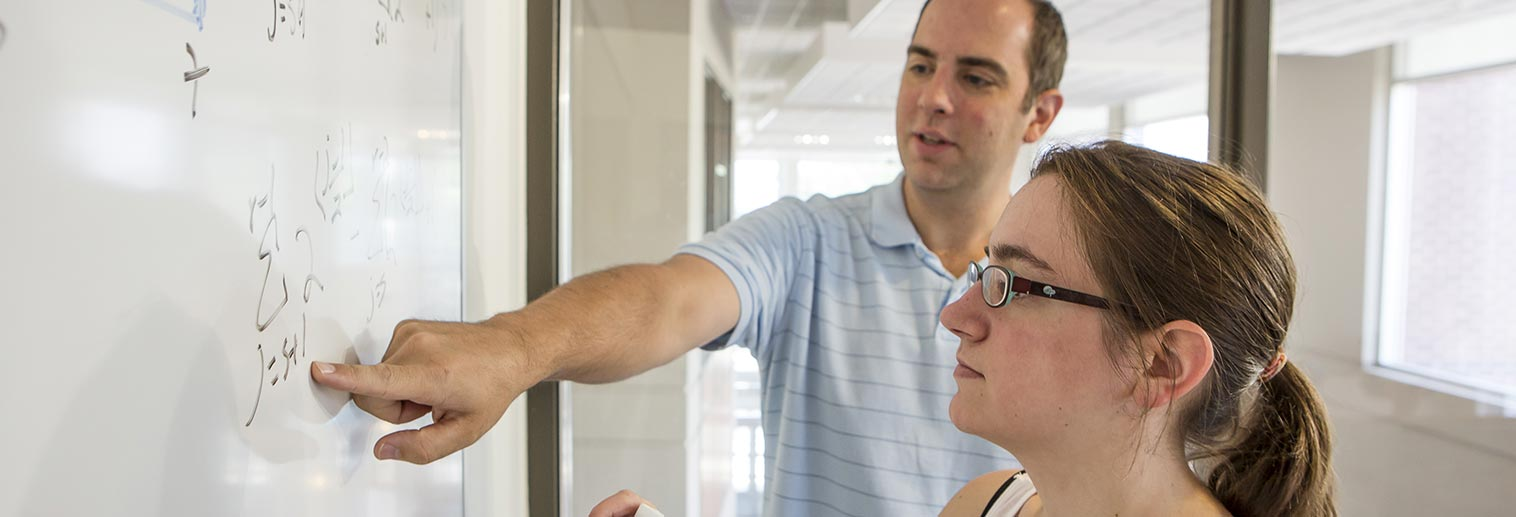# Programs of Study

## Bachelor of Science in Mathematics

Undergraduate mathematics majors at Moravian University benefit from a flexible curriculum with three track choices:

All mathematics majors are required to select a track when declaring their major.

## Pure Mathematics

For students who are seeking a broad mathematical background, Pure Mathematics provides the quantitative and analytical skills ideal for graduate school or education certification.

## Applied Mathematics

Students majoring in Applied Mathematics will develop the strong math foundation required to solve real-world problems. This is ideal for students interested in business, industry, or graduate school in applied mathematics.

## Actuarial Sciences

The Actuarial Sciences major prepares students to become actuaries, professionals who analyze the financial costs of risk and uncertainty. Students will be prepared to take Exam P and FM (the first two actuarial exams).

### Mathematics Minor

The minor in mathematics consists of five course units in mathematics:

• MATH 170 (or the equivalent sequence MATH 106 and MATH 166)
• MATH 171
• Three MATH courses numbered 210 or above

### Statistics Minor

Statistical analysis plays an important role in any evidence-based decision making. The statistics minor will provide students with a theoretical foundation of probability and statistics, an introduction to computing using statistical software, a foundation for applying statistical models to analyze data, and the skills to communicate their results.

The statistics minor is useful for non-mathematical majors because it provides students with a deeper understanding of statistics and quantitative reasoning. In addition to having students work with data and research questions from a variety of disciplines, the minor will emphasize the importance of evidence-based, data-driven decisions and foster skills for students to be critical observers in society—skills that complement and strengthen a range of majors and careers.

The minor in statistics consists of five courses: MATH 171, MATH 232, MATH 231, MATH 338, and one upper-level course that either focuses on the theory of statistical inference (MATH 337; recommended for those applying to graduate school in statistics) or on the application of statistics in a specific discipline.

### Curriculum Flexibility

The mathematics major curriculum allows students to pursue multiple majors and minors. Recent examples include:

### Looking for a related program?

Consider computer science